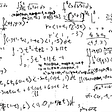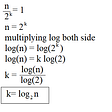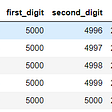# Fibonacci Sequence Exploration — Patterns, Proofs, Code

In this article we will explore various algorithms for calculating the nth Fibonacci term, derive formulas for various Fibonacci sums, and turn those formulas into programs.

If you are vaguely acquainted with mathematics it is likely that you have heard of the Fibonacci sequence. A particular term of the Fibonacci sequence is the sum of the previous two terms.

Using basic induction, we can derive expressions for the sums of Fibonacci terms.

# The Sum of the First N Fibonacci Terms

We will claim and prove that the sum of the first n terms of the Fibonacci sequence is equal to the sum of the nth term with the n+1th term minus 1.

# The Sum of First N Fibonacci Terms With Odd Indices

The sum of the first n Fibonacci terms with odd indices is the term located at 2n.

# The Sum of First N Fibonacci Terms With Even Indices

Now let’s use our previous two results to derive the first N terms with even indices. We can simply take the sum of the first 2n terms, and subtract the sum of the first n odd terms. The result will be the sum of the Fibonacci terms of even indices.

# Nth Fibonacci Term Coding Question

We will now explore four different programs; each of which return the nth Fibonacci term. We will begin with a basic recursive technique and build up to a constant time O(1) solution.

# Recursive Solution

A recursive solution is the most obvious implementation of the nth Fibonacci term as the sequence itself is a recurrence relation.

`def nth_fibonacci(n):  if (n <= 1):    return 1  else:    return nth_fibonacci(n - 1) + nth_fibonacci(n - 2)`

## Time Complexity Analysis

We can apply the master theorem on the recurrence relation; seeing that the second recursive call is approximately the same as the first recursive call, we get the following approximation of the time complexity.

# Memoization/Dynamic Programming Solution

One of the flaws in our recursive approach is that we will have repeat recursive calls. To demonstrate this, we will unwind the derived recurrence relation from above.

As you can see, for the first unwinding, we already have a redundant call. Just imagine how many redundant calls we accumulate for any sizeable n! To remedy this; we can use an altered approach where the computer remembers these redundant values so we do not need to recalculate them. This is known as memoization.

We can drastically improve our time complexity by using an array to store previous terms. We can then produce our current term by summing up two terms which have already been saved.

`def fib(self, n: int) -> int:	#Initialize first two terms    dp = [0,1]    if n < 2:        return dp[n]    for i in range(1,n):        dp.append(dp[i]+dp[i-1])    return dp[-1] `

In the code above; we initialize the first two terms of the Fibonacci sequence and iteratively build up to our desired term by saving previous ones.

## Time and Space Complexity Analysis

The time complexity of this algorithm is O(n) as we only need to perform n constant time calculations within our main loop. The space complexity is also O(n) as our array must store n terms.

# Space Efficient Fibonacci DP Solution

The above dynamic programming solution reduces the time complexity for the nth Fibonacci term; but is still memory inefficient. How can we reduce our space complexity? On any given calculation; we are only utilizing the calculations of the previous two terms. For that reason; we can get away with using an array of size 3 to calculate any Fibonacci term. We can simply reallocate terms as we iterate.

`def fib(self, n: int) -> int:    #initialize length 3 array    dp = [0,1,0]    if n == 0:         return dp    elif n == 1:        return dp    else:        for i in range(1,n):            dp = dp+dp            dp = dp            dp = dp    return dp`

The first two terms are base cases. The trick is that we calculate the current term and then allocate the second term as the first, and our current term as the second in the array. This prepares the array for the next calculation.

## Time and Space Complexity Analysis

The time complexity of this solution is still O(n), and the space complexity is now O(1) as we have a constant time array initialized.

# Constant Time Fibonacci Solution

It turns out there is an even faster method of calculation for the nth Fibonacci term. We will utilize Binet’s formula for the golden ratio to produce an O(1) time and space solution.

`def fib(self, n: int) -> int:    p = (1 + math.sqrt(5)) / 2    return round(p**n/math.sqrt(5))`

# Fibonacci Sums With Code

Using the first three theorems we proved above, and our new optimal solution for the nth Fibonacci term; we can write code which calculations the sums of the first n terms, the first n even indexed terms, and odd indexed terms all in constant time and space.

# First N Terms Sum

`def fib_sum(n):    return fib(n+2) - 1`

# First N Terms Sum Odd Indices

`def fib_odd_sum(n):    return fib(2*n)`

# First N Terms Sum Even Indices

`def fib_even_sum(n):	return fib(2*(n+1))-1`

Originally published at https://www.pranav.ai on November 14, 2021.

--

--

--

## More from Pranav Ahluwalia

Computer Scientist Blog: www.pranav.ai Website: www.prandev.com

Love podcasts or audiobooks? Learn on the go with our new app.

## Explore Math with Paper Snowflake Art## Explore Math at the Farmers’ Market## 22 Amazing Math Tools That Everyone Should Know But Only Very Few Know## Mathematics: A Zoo Story## September 11, 2008## 22 Wonderful Math Books for Lifelong Learners to Read in 2022## Pranav Ahluwalia

Computer Scientist Blog: www.pranav.ai Website: www.prandev.com

## Binary Exponentiation## Explicated Linear Algebra using NM Dev Libraries## PyTorch quick reference — Tensors## Multiplication Using Machine Learning